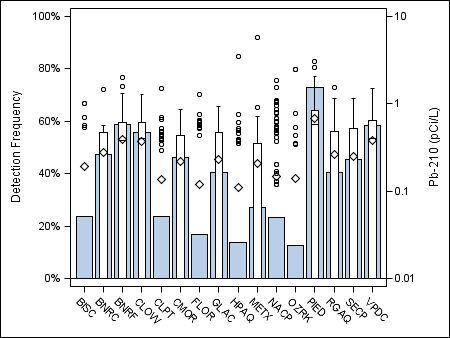## Control order of bars for a GTL barchart

How can you control the order of bars in a barchart created in GTL? I sorted the bar variable (percent) in descending order but the plot orders in the bars alphabetically be the group variable (prinaquifer2).

/*calculate detection frequency of Pb210 by category in percentage*/

proc freq data=b;

by PrinAquifer2;

tables H17503*PrinAquifer2 / noprint out=Freq1Out; /* save Percent variable */

run;

data Freq2Out; set Freq1Out;

if H17503=0 then delete;

Percent = Percent / 100;

format Percent PERCENT5.;

run;

proc sort data=Freq2Out; by descending Percent; run;

/*combine data sets*/

data plot;

set b Freq2Out;

keep PrinAquifer2 percent P17503;

run;

proc template;

define statgraph testplot;

begingraph;

layout overlay / Backgroundcolor=CXFFFFFF Border=False Wallcolor=CXFFFFFF Opaque=False

yaxisopts=(label="Detection Frequency" linearopts=(viewmin=0 viewmax=1 tickvaluesequence=(start=0 end=1 increment=.2)))

y2axisopts=(type=log label="Pb-210 (pCi/L)")

xaxisopts=(display=(line ticks tickvalues));

barchart x=PrinAquifer2 y=percent;

boxplot x=PrinAquifer2 y=P17503 / yaxis=y2 fillattrs=(color=CXFFFFFF) ;

endlayout;

endgraph;

end;

run;

ods graphics / width=450px;

ods listing;

*ods latex path="C:\NYBackup\projects\Glacial\Publications\Po210nPb210\Pb210";

*ods graphics on / imagefmt=pdf;

proc sgrender data=plot

template=testplot;

run;

*ods graphics off;

*ods latex close;

run; quit;5 REPLIES 5

## Re: Control order of bars for a GTL barchart

could try type=discrete
xaxisopts=(type=discrete discreteOpts=(tickValueList='PIED' 'RGAQ' 'SECP' '' ) display=(line ticks tickvalues));

Thanks,
Jag

## Re: Control order of bars for a GTL barchart

I see where you're going with this but TIckValueList=(numeric-list) so I don't think it will work with characters. I tried and got errors:

xaxisopts=(type=discrete discreteOpts=(tickValueList='PIED' 'BNRF'

---

346! 'VPDC' 'CLOW' 'BNRC' 'CMOR' 'SECP' 'GLAC' 'RGAQ' 'METX'

347 'BISC' 'CLPT' 'NACP' 'FLOR' 'HPAQ' 'OZRK') display=(line ticks

--- --- --- ---

49 49 49 49

347! tickvalues));

NOTE 49-169: The meaning of an identifier after a quoted string might change in a future SAS

release. Inserting white space between a quoted string and the succeeding

identifier is recommended.

## Re: Control order of bars for a GTL barchart

Since the online documentation explicitly shows this as an example:

`tickvaluelist=("Sedan" "Sports" "Wagon" "SUV")`

then the issue is not character values.

Also what you show is not an ERROR. It is a NOTE that somewhere you have a quote followed by a character other than a space. Since there are a number of syntax features such as date literals: '01JAN2017'd and 'names not valid'n in some SAS sessions the quote-character combination may be used for some use in the future.

When posting log results it is best to post the entire section of code, such as from Proc Template, as the actual issue sometimes starts lines before the indicated error, warning or note. And post into a code box opened using the Forum {i} menu icon to prevent the message window from reformatting text. Not that you message shows underscores that originally appeared under a different part of the code an almost certainly did not appear in the left margin.

Providing some data in the form of a data step would help to provide an actual tested solution.

## Re: Control order of bars for a GTL barchart

Jag,

Please accept my apologies. Your suggestion for controlling the order of bars for a GTL barchart did work. I revisited your post after recieving other feedback and using your code solved my problem. My initial reaction that tickValueList would only work with numerics was due to a coding error on my part.DanH_sas
SAS Super FREQ

## Re: Control order of bars for a GTL barchart

The best way to handle this is to format the numeric column to a string column. The numeric category values are automatically getting sorted in numeric order. On your BARCHART and BOXPLOT statements, try this and see if it works for you:

barchart x=eval(put(PrinAquifer2, BEST.)) y=percent;

boxplot x=eval(put(PrinAquifer2, BEST.)) y=P17503 / yaxis=y2 fillattrs=(color=CXFFFFFF) ;

Hope this helps!

Dan

Discussion stats
• 5 replies
• 1442 views
• 3 likes
• 4 in conversation